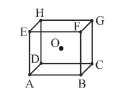Copper metal has a face-centred cubic structure with the unit cell length equal to 0.361 nm. Picturing copper ions in contact along the face diagonal. The apparent radius of a copper ion is-

(A) 0.128

(B) 1.42

(C) 3.22

(D) 4.22

Concept Questions :-

Density/Formula/packing fraction/ Semiconductors
High Yielding Test Series + Question Bank - NEET 2020

Difficulty Level:

Choose the correct matching sequence from the possibilities given

(a) Crystal defect                             (1) AB AB AB.....type crystal

(b) hcp                                            (2) Covalent crystal

(c) CsCl                                           (3) Frenkel

(d) Diamond                                    (4) Face centered in cube

(e) NaCl                                          (5) Body centered in cube
(a)           (b)               (c)             (d)                   (e)

(A)           3               1                 2                5                     4

(B)           3               1                 5                2                     4

(C)           3               5                 1                2                     4

(D)           5               3                4                 2                     1

Concept Questions :-

Imperfections in Solids
High Yielding Test Series + Question Bank - NEET 2020

Difficulty Level:

A compound alloy of gold and copper crystallizes in a cube lattice in which the gold atoms occupy the lattice points at the corners of a cube and the copper atoms occupy the centres of each of the cube faces. The formula of this compound is-

(A) AuCu

(B) $AuC{u}_{2}$

(C) $AuC{u}_{3}$

(D) None of these

Concept Questions :-

Density/Formula/packing fraction/ Semiconductors
High Yielding Test Series + Question Bank - NEET 2020

Difficulty Level:

Select the correct statement (s) -

(a) The C.N. of cation occupying a tetrahedral hole is 4.

(b) The C.N. of cation occupying a octahedral hole is 6.

(c) In schottky defects, density of the lattice decreases,

(A) a, b

(B) b, c

(C) a, b, c

(D) a, c

Concept Questions :-

Introduction and Type of Crystal System
High Yielding Test Series + Question Bank - NEET 2020

Difficulty Level:

Among the following types of voids, which one is the largest void-

(A) Triangular system

(B) Tetragonal system

(C) Monoclinic system

(D) Octahedral

Concept Questions :-

Introduction and Type of Crystal System
High Yielding Test Series + Question Bank - NEET 2020

Difficulty Level:

Bragg’s equation is-

(A) $n\lambda =2\theta \mathrm{sin}\theta$

(B) $n\lambda =2d\mathrm{sin}\theta$

(C) $2n\lambda =d\mathrm{sin}\theta$

(D) $\lambda =\left(2d/n\right)\mathrm{sin}\theta$

High Yielding Test Series + Question Bank - NEET 2020

Difficulty Level:

The rank of a cubic unit cell is 4. The type of cell as-

(A) Body centred

(B) Face centred

(C) Primitive

(D) None of these

Concept Questions :-

Introduction and Type of Crystal System
High Yielding Test Series + Question Bank - NEET 2020

Difficulty Level:

Close packing is maximum in the crystal which is-

(A) Simple cube

(B) bcc

(C) fcc

(D) None

Concept Questions :-

High Yielding Test Series + Question Bank - NEET 2020

Difficulty Level:

In a face centred cubic arrangement of metallic atoms, what is the relative ratio of the sizes of tetrahedral and octahedral voids ?

(A) 0.543

(B) 0.732

(C) 0.414

(D) 0.637

Concept Questions :-

High Yielding Test Series + Question Bank - NEET 2020

Difficulty Level:

A body centred cubic arrangement is shown : O is the body centre; A, B, C, D,......., H are the corners. What is the magnitude of the angle AOB ?(A) $120°$

(B) $109°28\text{'}$

(C) $104°31\text{'}$

(D) $70°32\text{'}$

Concept Questions :-

Density/Formula/packing fraction/ Semiconductors